## 1. Introduction

Printing is a very common visualization technique for data structures. It can be tricky when it comes to trees, though, due to their hierarchical nature.

In this tutorial, we'll learn some printing techniques for Binary Trees in Java.

## 2. Tree Diagrams

Despite the limitations of drawing with only characters over on console, there are many different diagram shapes to represent tree structures. Choosing one of them mostly depends on the size and the balance of the tree.

Let's take a look at some of the possible types of diagrams which we can print: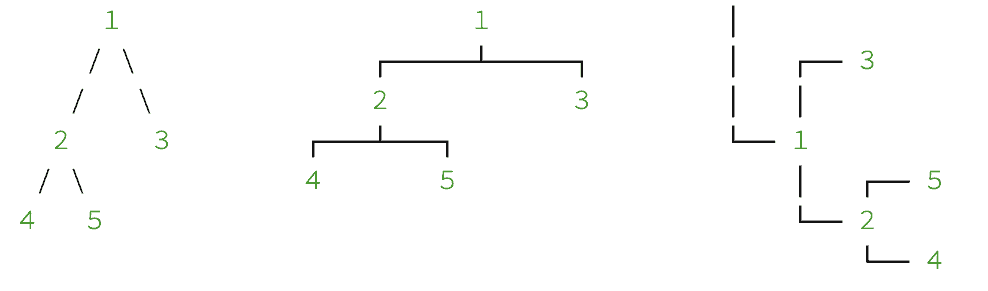But, we will explain a practical one which is also easier to implement. By taking the direction into account in which the tree grows, we can call it a horizontal tree: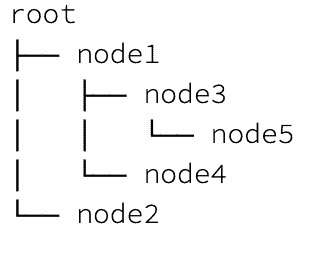Because the horizontal tree flows always in the same direction as the text flows, we have some benefits to choosing a horizontal diagram over others:

1. We can visualize large and unbalanced trees as well
2. The length of node values doesn't affect the display structure
3. It is much easier to implement

Therefore, we will go with the horizontal diagram and implement a simple binary tree printer class in the next sections.

## 3. Binary Tree Model

First of all, we should model a basic binary tree which we can do with just a few lines of code.

Let's define a simple BinaryTreeModel class:

``````public class BinaryTreeModel {

private Object value;
private BinaryTreeModel left;
private BinaryTreeModel right;

public BinaryTreeModel(Object value) {
this.value = value;
}

// standard getters and setters

}
``````

## 4. Sample Test Data

Before we start implementing our binary tree printer, we need to create some sample data to incrementally test our visualization:

``````BinaryTreeModel root = new BinaryTreeModel("root");

BinaryTreeModel node1 = new BinaryTreeModel("node1");
BinaryTreeModel node2 = new BinaryTreeModel("node2");
root.setLeft(node1);
root.setRight(node2);

BinaryTreeModel node3 = new BinaryTreeModel("node3");
BinaryTreeModel node4 = new BinaryTreeModel("node4");
node1.setLeft(node3);
node1.setRight(node4);

node2.setLeft(new BinaryTreeModel("node5"));
node2.setRight(new BinaryTreeModel("node6"));

BinaryTreeModel node7 = new BinaryTreeModel("node7");
node3.setLeft(node7);
node7.setLeft(new BinaryTreeModel("node8"));
node7.setRight(new BinaryTreeModel("node9"));``````

## 5. Binary Tree Printer

Certainly, we need a separate class to keep our BinaryTreeModel clean for the sake of Single Responsibility Principle.

Now, we could use the Visitor Pattern so that the tree handles the hierarchy and our printer just handles the printing. But for this tutorial, we'll keep them together in order to keep it simple.

Thus, we define a class named BinaryTreePrinter and start implementing it.

### 5.1. Pre-Order Traversal

Considering our horizontal diagram, to print it properly, we can make a simple start by using pre-order traversal.

Consequently, to perform pre-order traversal, we need to implement a recursive method that first visits the root node, then left subtree, and finally the right subtree.

Let's define a method to traverse our tree:

``````public void traversePreOrder(StringBuilder sb, BinaryTreeModel node) {
if (node != null) {
sb.append(node.getValue());
sb.append("\n");
traversePreOrder(sb, node.getLeft());
traversePreOrder(sb, node.getRight());
}
}
``````

Next, let's define our print method:

``````public void print(PrintStream os) {
StringBuilder sb = new StringBuilder();
traversePreOrder(sb, this.tree);
os.print(sb.toString());
}
``````

Thus, we can simply print our test tree:

``````new BinaryTreePrinter(root).print(System.out);
``````

The output will be the list of tree nodes in traversed order:

``````root
node1
node3
node7
node8
node9
node4
node2
node5
node6
``````

To set up our diagram correctly, we use three types of characters “├──”, “└──”, and “│” to visualize nodes. The first two of them are for pointers and the last one is to fill the edges and connect the pointers.

Let's update our traversePreOrder method, add two parameters as padding and pointer, and use the characters respectively:

``````public void traversePreOrder(StringBuilder sb, String padding, String pointer, BinaryTreeModel node) {
if (node != null) {
sb.append(pointer);
sb.append(node.getValue());
sb.append("\n");

String pointerForRight = "└──";
String pointerForLeft = (node.getRight() != null) ? "├──" : "└──";

}
}
``````

Also, we update print method as well:

``````public void print(PrintStream os) {
StringBuilder sb = new StringBuilder();
traversePreOrder(sb, "", "", this.tree);
os.print(sb.toString());
}
``````

So, let's test our BinaryTreePrinter again: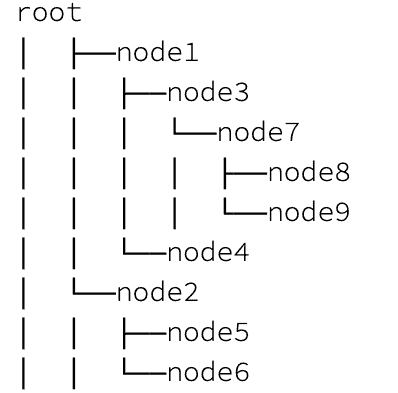Thus, with all the paddings and pointers, our diagram has shaped up nicely.

However, we still have some extra lines to get rid of: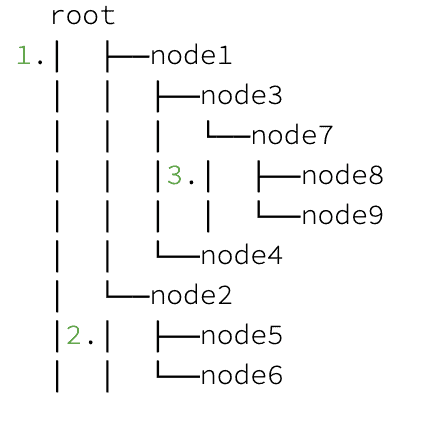As we look over on diagram, there are still characters in three wrong places:

1. The column of extra lines under the root node
2. The extra lines under the right subtree
3. The extra lines under the left subtree which has no right sibling

### 5.3. Different Implementations for Root and Child Nodes

In order to fix extra lines, we can split up our traverse method. We'll apply one behavior to the root node and another for child nodes.

Let's customize traversePreOrder for only the root node:

``````public String traversePreOrder(BinaryTreeModel root) {

if (root == null) {
return "";
}

StringBuilder sb = new StringBuilder();
sb.append(root.getValue());

String pointerRight = "└──";
String pointerLeft = (root.getRight() != null) ? "├──" : "└──";

traverseNodes(sb, "", pointerLeft, root.getLeft(), root.getRight() != null);
traverseNodes(sb, "", pointerRight, root.getRight(), false);

return sb.toString();
}
``````

Next, we'll create another method for child nodes as traverseNodes. Additionally, we will add a new parameter hasRightSibling to implement the preceding lines correctly:

``````public void traverseNodes(StringBuilder sb, String padding, String pointer, BinaryTreeModel node,
boolean hasRightSibling) {
if (node != null) {
sb.append("\n");
sb.append(pointer);
sb.append(node.getValue());

if (hasRightSibling) {
} else {
}

String pointerRight = "└──";
String pointerLeft = (node.getRight() != null) ? "├──" : "└──";

traverseNodes(sb, paddingForBoth, pointerLeft, node.getLeft(), node.getRight() != null);
}
}
``````

Also, we need a small change in our print method:

``````public void print(PrintStream os) {
os.print(traversePreOrder(tree));
}
``````

Finally, our diagram has formed into a nice shape with a clean output: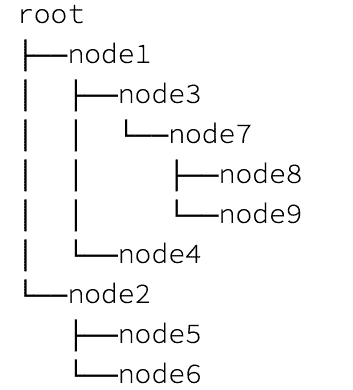## 6. Conclusion

In this article, we learned a simple and practical way to print out a Binary Tree in Java.